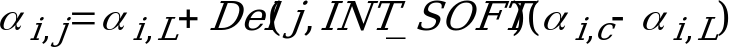## 4.1.6 Soft interpolation of the spectral parameters

06.203GPPHalf rate speech transcodingTS

Interpolation of the short term filter parameters improves the performance of the GSM half rate encoder. The direct form filter coefficients (i’s), which correspond to quantized reflection coefficients, are the spectral parameters used for interpolation. The GSM half rate speech encoder uses either an interpolated set of i’s or an uninterpolated set of i’s, choosing the set which gives better prediction gain for the frame.

Two sets of LPC coefficient vectors are generated: the first corresponds to the interpolated coefficients, the second to the uninterpolated coefficients. The frame’s speech samples are inverse filtered using each of the two coefficient sets, and the residual frame energy corresponding to each set is computed. The coefficient set yielding the lower frame residual energy is then selected to be used. If the residual energies are equal, the uninterpolated coefficient set is used. INT_LPC, a soft interpolation bit, is set to 1 when interpolation is selected or to 0 otherwise.

To generate the interpolated coefficient set, the coder interpolates the i’s for the first, second, and third subframes of each frame. The fourth subframe uses the uninterpolated i’s for that frame.

The interpolation is done as follows. Let i,L be the direct-form LPC coefficients corresponding to the last frame, i,C be the direct-form LPC coefficients corresponding to the current frame, and Del to be the interpolation curve used. The interpolated direct-form LPC coefficient vector at the j-th subframe of the current frame, i,j, is given by:, 1 £ i £ Np, 1 £ j £ 4 (31)

The values of the interpolation curve Del are given in table 2.

Table 2: Values of the interpolation curve Del

 j Del(j,0) Del(j,1) 1 0,0 0,30 2 1,0 0,62 3 1,0 0,92 4 1,0 1,00

From this point on, the subframe index j is omitted for simplicity when referring to i,j coefficients, although it is implied. For interpolated subframes, the i’s are converted to reflection coefficients to check for filter stability. If the resulting filter is unstable, then uninterpolated coefficients are used for that subframe. The uninterpolated coefficients used for subframe 1 are the previous frame’s coefficients. The uninterpolated coefficients used for subframes 2, 3, and 4 are the current frame’s coefficients.